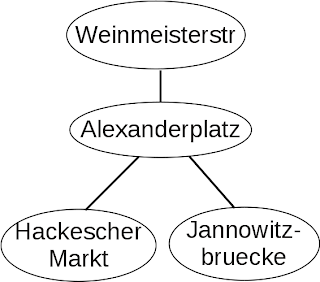## Adjacency matrix and topology of graph

Last time, I explained about what is an adjacency matrix. This time I would like to show the relationships between an adjacency matrix and the corresponding graph.

An adjacency matrix shows how the nodes are connected. When we care only the existence of connection, we call such mathematics topology. Sometimes only the connection is important even in every day life. For example, we usually only care the city train connection, the real distance on the map is less important. Two stations might be less than 300m away, but if there is no train connection between these two stations, sometimes you think these trains are totally separated stations. The map of  Liniennetz found in the web site of Berlin city transportation is such example. You can see the ring-bahn as a nearly perfect ellipse on the map, but, if you check the real map, it is not the same shape. The train map is focusing on the connection between stations. This is a kind of topology map.Figure 7: Graph example 2. Each node is a train station.
This is a small part of Berlin train network. You can see three train stations connected to Alexanderplatz station. The adjacency matrix of this graph is the following. (We only use first four letters of the train stations here.)

\begin{eqnarray*}
\begin{array}{ccccc}
& \mbox{Wein.} & \mbox{Alex.} & \mbox{Hack.} & \mbox{Jann.} \\
\begin{array}{c}
\\
\mbox{Wein.}\\
\mbox{Alex.}\\
\mbox{Hack.}\\
\mbox{Jann.}\\
\end{array}
&
\left[
\begin{array}{c}
0 \\
1 \\
0 \\
0 \\
\end{array}
\right.
&
\begin{array}{c}
1\\
0\\
1\\
1\\
\end{array}
&
\begin{array}{c}
0\\
1\\
0\\
0\\
\end{array}
&
\left.
\begin{array}{c}
0\\
1\\
0\\
0\\
\end{array}
\right]
\end{array}
\end{eqnarray*}

The number of 1s of row vector tells us how many edges are connected to the node. For example, the row vector of Weinmeisterstr is the following.

\begin{eqnarray*}
\begin{array}{ccccc}
\begin{array}{c}
\mbox{Wein.}
\end{array}
&
\left[
\begin{array}{c}
0 \\
\end{array}
\right.
&
\begin{array}{c}
1\\
\end{array}
&
\begin{array}{c}
0\\
\end{array}
&
\left.
\begin{array}{c}
0\\
\end{array}
\right]
\end{array}
\end{eqnarray*}

The number pf 1s is 1, therefore, the number of edges from Weinmeisterstr node is 1. This number is called degree'' of the node. Alexanderplatz row vector has three 1s as shown in below, therefore, the degree of Alexanderplatz node is 3.

\begin{eqnarray*}
\begin{array}{ccccc}
\begin{array}{c}
\mbox{Alex.}
\end{array}
&
\left[
\begin{array}{c}
1 \\
\end{array}
\right.
&
\begin{array}{c}
0\\
\end{array}
&
\begin{array}{c}
1\\
\end{array}
&
\left.
\begin{array}{c}
1\\
\end{array}
\right]
\end{array}
\end{eqnarray*}

You can check the degree of nodes also in Figure 7.

Next time I would like to show you some operations of the matrix.